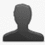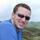This project is archived and is in readonly mode.

✓invalid## Number round and addition problem ?

Reported by grazius | September 30th, 2009 @ 01:49 PM | in 2.0 (closed)

Hello,
why i've got that :

(52.27111111).round(2) = 52.27

(26.80111111).round(2) = 26.8

(26.80111111).round(2) + (52.271111).round(2) = 79.07000000000001

and need to do that to get the right value ?
((26.80111111).round(2) + (52.271111).round(2)).round(2) = 79.07

Strange bug or not ?

### Comments and changes to this ticket

•#### David CandrevaSeptember 30th, 2009 @ 05:23 PM

I'm not understanding what your bug is?

You can do:

``````var one = 52.27111111;
var two = 26.80111111;

var total = (one + two).round(2); // returns 79.07```
```

or

````var total = (52.27111111 + 26.8011111).round(2); // also returns 79.07`
```
•#### Scott KyleOctober 2nd, 2009 @ 01:07 AM

• State changed from “new” to “invalid”

Welcome to the world of floating point math on computers. :-)

David's suggestion of rounding at the last step is correct.

For more info on why floating point arithmetic can't be 100% accurate on computers, I recommend googling the subject.

•#### graziusOctober 3rd, 2009 @ 09:19 AM

Hello,

The rouding of the sum is a different operation as the sum of rounding values.

(52.995).round(2) + (26.995).round(2) 80
(52.995 + 26.995).round(2) 79.99

I know this problem for a long time in java and i see in javascript they no exist simple alternative like bigDecimal object.

•#### graziusOctober 3rd, 2009 @ 09:33 AM

best example

(10.018).round(2) + (10.996).round(2) 21.02
(10.018 + 10.996).round(2) 21.01

•#### Fábio M. CostaOctober 3rd, 2009 @ 03:30 PM

Grazius, your example is correct, it should give e different results.

(10.018).round(2) + (10.996).round(2):

(10.018).round(2) this results in 10.2, which is the correct behavior;

(10.996).round(2) => 11, correct too.

10.2 + 11 // 21.02

(10.018 + 10.996).round(2):

10.018 + 10.996 => 21.014, which rounded gives 21.01, correct!